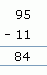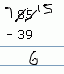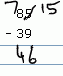Name: ___________________Date:___________________

kwizNET Subscribers, please login to turn off the Ads!
 Email us to get an instant 20% discount on highly effective K-12 Math & English kwizNET Programs!

### Grade 1 - Mathematics3.22 Subtraction - Double Digit

Subtraction without borrow
Method:
1. Start from the units place
2. Subtract the numbers in the units place
3. Subtract the numbers in the tens place

Example:
 Question Step 1 Step 2Subtraction with borrow
Method:
1. Look at the numbers in the units.
2. If the number on the bottom is more than the number at the top. Borrow 1 from the tens place.
3. Decrease 1 from the tens place and add 10 to units place.
4. Now perform subtraction for the units place.
5. Look at the numbers in the tens place
6. Perform similar subtraction for the tens place
Example:

 Question Step 1 Step 2The units digits, 5 is less than 9, therefore borrow 1 from tens place that is from 8.
Decrease 1 from 8 and add 10 to units place to become 15.
Subtract 15 and 9 to get 6.
Subtract 7 and 3 to get 4.

Answer: 46

Directions: Answer the following questions. Also write at least ten examples for subtraction without borrow and subtraction with borrow of your own.Name: ___________________Date:___________________

### Grade 1 - Mathematics3.22 Subtraction - Double Digit

 Question 1: 90 - 57
Answer:

 Question 2: 68 - 21
Answer:

 Question 3: 36 - 22
Answer:

 Question 4: 84 - 53
Answer:

 Question 5: 31 - 24
Answer:

Question 6: This question is available to subscribers only!

Question 7: This question is available to subscribers only!

Question 8: This question is available to subscribers only!

Question 9: This question is available to subscribers only!

Question 10: This question is available to subscribers only!

#### Subscription to kwizNET Learning System costs less than \$1 per month & offers the following benefits:

• Unrestricted access to grade appropriate lessons, quizzes, & printable worksheets
• Instant scoring of online quizzes
• Progress tracking and award certificates to keep your student motivated
• Unlimited practice with auto-generated 'WIZ MATH' quizzes
• Child-friendly website with no advertisements

© 2003-2007 kwizNET Learning System LLC. All rights reserved. This material may not be reproduced, displayed, modified or distributed without the express prior written permission of the copyright holder. For permission, contact info@kwizNET.com
For unlimited printable worksheets & more, go to http://www.kwizNET.com.